Next: 6.2.5 Debye-Hückel Model Up: 6.2 Modeling BioFETs Previous: 6.2.3 Poisson-Boltzmann Model with

## 6.2.4 Extended Poisson-Boltzmann Model

The extended Poisson-Boltzmann model  incorporates the average closest possible distance two ions within the liquid can approach. This allows to include the Stern layer within this formulation without the need to add an ion free zone between the dielectric and the region where the Poisson Boltzmann model is working. Furthermore, the closest possible approach between two ions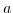is in this model a fit parameter and can therefore account for the varying screening behavior at different ionic concentrations: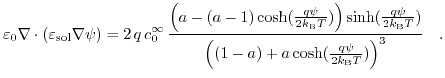(6.5)

Here,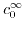denotes the bulk ion concentration for a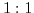salt. In the limit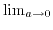the Poisson Boltzmann expression is recovered. One has to mention that this formulatin is limited toelectrolytes and therefore can not be applied to an arbitrary solute. In Section 6.3 some examples will be considered.Next: 6.2.5 Debye-Hückel Model Up: 6.2 Modeling BioFETs Previous: 6.2.3 Poisson-Boltzmann Model with

T. Windbacher: Engineering Gate Stacks for Field-Effect Transistors